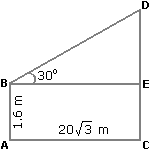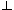# Aptitude - Height and Distance - Discussion

### Discussion :: Height and Distance - General Questions (Q.No.4)

4.

An observer 1.6 m tall is 203 away from a tower. The angle of elevation from his eye to the top of the tower is 30°. The height of the tower is:

 [A]. 21.6 m [B]. 23.2 m [C]. 24.72 m [D]. None of these

Explanation:

Let AB be the observer and CD be the tower.Draw BECD.

Then, CE = AB = 1.6 m,

BE = AC = 203 m.

 DE = tan 30° = 1 BE 3DE = 203 m = 20 m. 3CD = CE + DE = (1.6 + 20) m = 21.6 m.

 Yazhini.M.R said: (Aug 3, 2011) Since tan thetq= opposite/adjacent , tan 30= DE/BE , the value of tan 30 is 1/ root 3, then DE/BE=1/root 3, then DE=BE/root 3, thus 20 m is obtained. since CD= CE+DE, 21.6 is obtained.

 Anurag said: (Sep 19, 2016) Here DE/BE = 1/root3. So, DE will be cross multiply by roo3 and BE will be multiplied by 1. So, how come the value of DE is divided by root 3?

 Priya58310 said: (Nov 13, 2016) Why they applied ''tan" what is the rule behind that?

 Ravina said: (Feb 9, 2017) I too had the Same doubt @Priya. Please clear the doubt of as.

 Jatin007 said: (Feb 11, 2017) Applying tan because of we need to find DE. So tan 30= DE/BE. tan 30=DE/20√3, DE=20√3*tan30( value of tan 30=1/√3), So DE= 20. So CD= EC+ED=1.6+20=21.6(answer).

 Mahksh said: (Feb 13, 2017) There is no specific rule for this. According to the situation we have to find this. Here, in this case, DE/BE i.e. perpendicular upon base means "tan". So we take tan 30.

 Monu_Yadav said: (Mar 15, 2017) But the unit of 20√3 is not given in a problem. So,answer is None of these.

 Akshaya Prasad Sahoo said: (Sep 22, 2017) Why are you using tan 30?

 Lakshaya said: (Oct 2, 2017) Why be is Perpendicular to cd?

 Seeli said: (Nov 5, 2017) Why they not use cos 30?

 Rocky said: (Jun 29, 2018) The correct answer is 61.6 so none of these is the correct option.

 Midhun said: (Oct 1, 2018) @Akshaya Prasad. We use tan, sin or cos depending upon what we want to get and what they have given in the question. For example. If they give opposite side and you want to find the adjacent side. So, we will use a tan.

 Akshatha said: (Oct 9, 2018) @Rocky. Yes, it is the right answer.

 Dipakk said: (Jul 30, 2019) @Jatin. Why EC & ED added?

 Kesavan said: (Aug 2, 2019) I'm not getting whether to use tan or cos. Please, anyone, clarify it in detail.

 Pooja Kale said: (Oct 15, 2019) Remember basic rule, If Finding : Perpendicular : Sin Hypotenuse : cos Base : tan